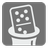Magic Domino
Challenging
English RU

When Nikola can not find opponent for a game of Dominoes, he spreads them out for a game of Magic Domino Solitaire. The goal of solitaire is to build a Magic square with Double Six domino tiles.

A magic square is an arrangement of distinct integers, in a square grid, where the numbers in each row, and in each column, and the numbers in the forward and backward main diagonals, all add up to the same number.
Domino tiles contain two numbers from 0 (empty) to 6. Tiles are unordered and 1-6 is the same as 6-1. The double-six set contains 28 unique tiles - all combinations of number pairs.You are given a size for the magic square and a number. You should build a magic square to the given size so that the sum of the horizontal and vertical diagonals equal the given number. You can place domino tiles from double-six set only vertically and they should be unique.

Here is example for size = 4 and number = 5:The result...

You should be an authorized user in order to see the full description and start solving this mission.